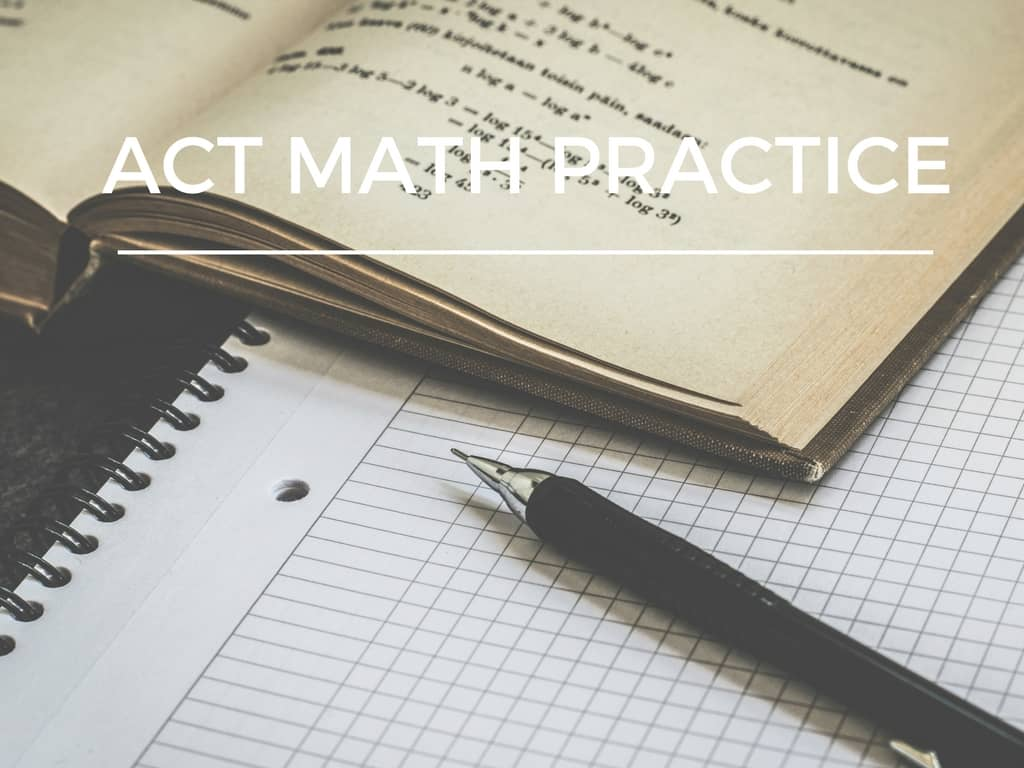Back to posts

# ACT Math PracticeFeeling nervous about the math portion of the ACT? You’re not alone! I have put together a study guide for you to use during your ACT Math practice sessions. You can use this with your tutor to identify your weak spots so you know where to focus. With that in mind, let’s start going over what information you need to know for successful ACT math practice.

What Do You Need to Know for ACT Math Practice?

Because the goal of the ACT is to measure your college readiness, you’re supposed to be well-prepared for it just by taking a good, college preparatory class schedule. And while that’s comforting to know, it’s not really helpful if math isn’t your best subject or if you’re struggling with this section during your ACT math practice sessions.

In order to help you better prepare for the Math section, I’ve compiled a list of the things you will absolutely need to know.

Numbers

•    Basic integers
•    identifying integers
•    differences between positive and negative integers
•    add, subtract, multiply, and divide both positive and negative integers
•    prime numbers
•    multiplying and dividing exponents and bases
•    square roots
•    solving the absolute value
•    consecutive numbers
•    remainders
•    Fractions
•    express whole numbers as fractions
•    0 divided by any number is 0
•    any number divided by 0 is undefined
•    reducing fractions
•    add, subtract, multiply, and divide fractions
•    expressing decimals as fractions
•    percentages as fractions
•    mixed fractions
•    Ratios
•    identify ratios
•    reducing and increasing ratios
•    finding the whole
•    Rational and Irrational Numbers
•    identify rational and irrational numbers
•    Proportions
•    Mean, median, mode, and statistics
•    identify mean, median, and mode
•    unweighted and weighted averages
•    Probabilities
•    define and express probabilities
•    either/or probability
•    combined probability
•    simple probability
•    probability ratio
•    altering probability
•    Sequences
•    arithmetic and geometric sequences
•    arithmetic sequence formulas like terms and sums formula
•    geometric sequence formula

Algebra

•    Equations with a single variable
•    identify a variable, equation, and a single variable equation
•    solve for x
•    Systems of Equations
•    solve equations by graphing, substitution, and subtraction
•    identify when equations have one, infinite, or no solutions
•    Operations
•    identify mathematical operations
•    identify equivalent expressions
•    Functions
•    identify linear and quadratic functions and their formulas
•    vertical line test
•    identify input, output, and ordered pairs

Coordinate Geometry

•    Points
•    distance formula
•    pythagorean formula
•    midpoint formula
•    Lines and Slopes
•    identify lines and slopes
•    rise over run
•    positive slope, negative slope, straight line, perpendicular lines, and parallel lines
•    find slope and equation of a line
•    Reflections
•    line of symmetry
•    Translations
•    how to notate translations and find final coordinates
•    Rotations
•    center point
•    positive vs negative rotation
•    rules and formulas for rotations

Plane and Solid Geometry

•    Lines and angles
•    identify parallel lines, perpendicular lines, equal angles, supplementary angles, opposite angles, and opposite interior angles
•    Circles
•    Triangles
•    identify parts of a triangle
•    identify equilateral triangles, isosceles triangles, right triangles, isosceles right triangle, 30-60-90 triangles, 3-4-5 triangles, and 5-12-13 right triangles
•    know formulas for area, perimeter, pythagorean theorem, isosceles right triangle, and 30-60-90 triangle
•    Polygons
•    identify irregular and regular polygons
•    identify quadrilaterals including parallelograms, rhombus, rectangle, square, trapezoid, and kite
•    know how to find area of a triangle, square, parallelogram, and trapezoid
•    know how to find interior angle sum with the interior angle formula or dividing polygons into triangles
•    Solid Geometry
•    identify spheres, prisms, cubes, cylinders, cones, pyramids, inscribed solids, and rectangular solids
•    know formulas for volume, surface area, and diagonal length for the above forms

Trigonometry

•    find sine, cosine and tangent of a right triangle
•    identify cosecant, secant, and cotangent of right triangle
•    find period of a graph
•    know laws of sines and cosines
•    identify which sides are opposite and adjacent
•    identify sine, cosine, tangent, period, and amplitude on graphs

There are about 31 formulas you should know when you take the ACT math section. Check out this link for the formulas and for a free study session to help you master them.

ACT Math Practice Materials

So now that you know what will be covered on the math section, let’s go over where you can find study materials to help prepare you for when you sit down to take the exam.

## Free ACT Math Practice Materials

Who doesn’t love free stuff? The good news is you don’t have to spend a fortune obtaining quality practice materials. Here are some ideas for getting access to free test prep materials.

The Official ACT Website

In addition to signing up for the ACT and getting your results online, the official ACT site also provides you with 60 sample math questions. With each question comes feedback on each answer choice to help you understand why the option is either correct or incorrect.

ACT Practice Tests

Because these give you an authentic test-taking experience, you need to add them to your ACT math practice materials. This will help you prepare the best for the ACT. Below, I’ve provided links to the available practice tests.